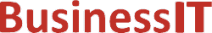O nás     Inzerce     KontaktSpolehlivé informace o IT již od roku 2011[Linux manuál]

# remainder, remainderf, remainderl: zbývající funkce

Originální popis anglicky: remainder, remainderf, remainderl - remainder function

Návod, kniha: POSIX Programmer's Manual

## STRUČNĚ

#include <math.h>

double remainder(double x, double y);

float remainderf(float x, float y );

long double remainderl(long double x, long double y);

## POPIS / INSTRUKCE

These functions shall return the floating-point remainder r= x- ny when y is non-zero. The value n is the integral value nearest the exact value x/ y. When | n-x/y|=0.5, the value n is chosen to be even.
The behavior of remainder() shall be independent of the rounding mode.

## NÁVRATOVÁ HODNOTA

Upon successful completion, these functions shall return the floating-point remainder r= x- ny when y is non-zero.
If x or y is NaN, a NaN shall be returned.
If x is infinite or y is 0 and the other is non-NaN, a domain error shall occur, and either a NaN (if supported), or an implementation-defined value shall be returned.

## CHYBY / ERRORY

These functions shall fail if:
Domain Error
The x argument is ±Inf, or the y argument is ±0 and the other argument is non-NaN.
If the integer expression (math_errhandling & MATH_ERRNO) is non-zero, then errno shall be set to [EDOM]. If the integer expression (math_errhandling & MATH_ERREXCEPT) is non-zero, then the invalid floating-point exception shall be raised.

The following sections are informative.

None.

## APPLICATION USAGE

On error, the expressions (math_errhandling & MATH_ERRNO) and (math_errhandling & MATH_ERREXCEPT) are independent of each other, but at least one of them must be non-zero.

None.

None.

## SOUVISEJÍCÍ

abs() , div() , feclearexcept() , fetestexcept() , ldiv() , the Base Definitions volume of IEEE Std 1003.1-2001, Section 4.18, Treatment of Error Conditions for Mathematical Functions, <math.h> Portions of this text are reprinted and reproduced in electronic form from IEEE Std 1003.1, 2003 Edition, Standard for Information Technology -- Portable Operating System Interface (POSIX), The Open Group Base Specifications Issue 6, Copyright (C) 2001-2003 by the Institute of Electrical and Electronics Engineers, Inc and The Open Group. In the event of any discrepancy between this version and the original IEEE and The Open Group Standard, the original IEEE and The Open Group Standard is the referee document. The original Standard can be obtained online at http://www.opengroup.org/unix/online.html .
 2003 IEEE/The Open Group
©2011-2020 BusinessIT.cz, ISSN 1805-0522 | Názvy použité v textech mohou být ochrannými známkami příslušných vlastníků.
Provozovatel: Bispiral, s.r.o., kontakt: BusinessIT(at)Bispiral.com | Inzerce: Best Online Media, s.r.o., zuzana@online-media.cz
O vydavateli | Pravidla webu BusinessIT.cz a ochrana soukromí | pg(8061)